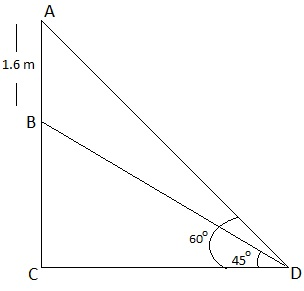# A statue, 1.6 m tall, stands on the top of a pedestal. From a point on the ground, the angle of elevation of the top of the statue is 60° and from the same point the angle of elevation of the top of the pedestal is 45°. Find the height of the pedestal.

Let AB be the height of statue.

D is the point on the ground from where the elevation is taken.

To Find: Height of pedestal = BC = AC-ABFrom the figure AB = statue = 1.6m

BC = pedestal = ?

In rt ∆ACD

P/B=AC/CD = tan60°

1.6 + BC/CD = √3

√3 CD = 1.6 + BC

CD = 1.6+BC/√3 -(1)

In rt ∆BCD,

BC/CD = tan45°

BC/CD = 1/1

CD = BC

From (1)

1.6 + BC/√3 = BC/1

√3 BC = 1.6 + BC

1.732 BC – 1 BC = 1.6

0.732 * BC = 1.6

BC = 1.6/0.732

BC = 16/10 * 100/732 = 1600/732

BC = 2.18m

Height of pedestal is 2.18m